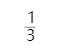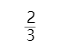HR
【多选题】毕达哥拉斯学派研究包括（ ）。
A.

B.

C.

D.手机使用分享复制链接新浪微博分享QQ微信扫一扫反馈MAN原则中，N（NEED），代表“需求”。这里的需求与经济学中的需求是同一概念（）
A.

B.

8 质量为$$m$$的小孩站在半径为$$R$$的水平转台边缘。转台可以绕通过其中心的竖直光滑固定轴自由转动，转动惯量为$$J$$。平台和小孩开始时均静止，当小孩突然以相对于地面为 $$\upsilon$$ 的速率在台边缘沿逆时针转向走动时，则此平台相对地面旋转的角速度和旋转方向分别为
A.
$$\omega =$$$$\frac{mR^2}{J}$$$$\centerdot$$($$\frac{\upsilon\ }{R})$$，顺时针
B.
$$\omega =$$$$\frac{mR^2}{J}$$$$\centerdot$$($$\frac{\upsilon\ }{R})$$，逆时针
C.
$$\omega =$$$$\frac{mR^2}{J+MR^2}$$$$\centerdot$$($$\frac{\upsilon\ }{R})$$，顺时针
D.
$$\omega =$$$$\frac{mR^2}{J+MR^2}$$$$\centerdot$$($$\frac{\upsilon\ }{R})$$，逆时针

A.

B.

10 光滑的水平桌面上，有一长为$$2L$$、质量为$$m$$的匀质细杆，可绕过其中点且垂直于杆的竖直光滑固定轴$$O$$自由转动，其转动惯量为$$mL^2/3$$，起初杆静止，桌面上有一个质量为$$m$$的小球，在杆的垂直方向正对着杆的一端以速率$$\upsilon$$运动，如图所示。 当小球与杆的端点发生完全非弹性碰撞后，就与杆粘在一起运动，则这一系统碰撞后的转动角速度为
A.
$$L\upsilon /12$$
B.
$$2\upsilon /(3L)$$
C.
$$3 \upsilon /(4L)$$
D.
$$3\upsilon /L$$

A.

B.

A.

B.

A.

B.

1 两个均质圆盘$$A$$和$$B$$密度分别为$$\rho_A$$和$$\rho_B$$，若$$\rho_A$$>$$\rho_B$$，但两圆盘质量与厚度相同，如两盘对通过盘心垂直于盘面轴的转动惯量分别为$$J_A$$和$$J_B$$，则
A.
$$J_A$$大于$$J_B$$
B.
$$J_A$$小于$$J_B$$
C.
$$J_A$$等于$$J_B$$
D.

A.B.2 一轻绳绕在具有水平转轴的定滑轮上，绳下端挂一物体，物体的质量为$$m$$，此时滑轮的角加速度为$$\alpha_A$$；若将物体取下，而用大小等于$$mg$$，方向向下的力拉绳子，此时滑轮的角加速度为$$\alpha_B$$
A.
$$\alpha_A$$等于$$\alpha_B$$
B.
$$\alpha_A$$大于$$\alpha_B$$
C.
$$\alpha_A$$小于$$\alpha_B$$
D.

A.

B.

A.

B.

555的6脚TH的作用时，当第5脚悬空时，此引脚电压升至（）Vcc时，输出端输出低电平。
A.
1/3
B.
2/3
3 一圆盘绕过盘心且与盘面垂直的光滑固定轴$$O$$以角速度$$\omega$$按图示方向转动。若如图所示的情况那样，将两个大小相等方向相反但不在同一条直线的力$$F$$沿盘面同时作用到圆盘上，则圆盘的角速度 $$\omega$$
A.

B.

C.

D.

A.

B.

C.

D.

A.

B.

4 一匀质细杆长为$$l$$，质量为$$m$$，杆两端用线吊起，保持水平。现有一条悬线突然断开，则断开瞬间另一条线的张力为
A.
$$3mg/4$$
B.
$$mg/4$$
C.
$$mg/2$$
D.
$$mg$$

A.

B.

C.

D.

A.

B.

A.

B.

C.

A.

B.

A.

B.

A.

B.

C.

D.

A.

B.

1 均匀细棒$$OA$$可绕通过其一端$$O$$而与棒垂直的水平固定光滑轴转动，现使棒从水平位置由静止开始自由下落，在棒摆动到竖直位置的过程中，下列说法哪一种是正确的
A.

B.

C.

D.

A.

B.

A.

B.

A.

B.

2 如图所示，一匀质细杆可绕通过上端与杆垂直的水平光滑固定轴$$O$$旋转，初始状态为静止悬挂．现有一个小球自左方水平打击细杆．设小球与细杆之间为非弹性碰撞，则在碰撞过程中对细杆与小球这一系统
A.

B.

C.

D.

A.
R1 R2 C R1 R2
B.
R1R2 R1R2C

A.

B.

A.

B.

C.

D.

A.

B.

C.

D.

3 一个圆柱体在水平面上以速率$$v$$做无滑动的滚动，要使它静止的话，需要做的功为
A.
$$\frac{1}{4}mv^2$$
B.
$$\frac{1}{2}mv^2$$
C.
$$\frac{3}{4}mv^2$$
D.
$$mv^2$$
E.
$$\frac{5}{4}mv^2$$

A.

B.

A.

B.

C.

D.

A.
B.
C.

D.

A.

B.

4 卡车沿平直轨道以恒定加速度$$a$$运动。为了测量车的加速度，从卡车天花板上垂挂一质量为$$m$$的均匀细长杆，若细长杆与铅直方向的夹角为$$\theta$$ ，则$$a$$与$$\theta$$ 的关系式为
A.
sin$$\theta =\frac{a}{m}$$
B.
cos$$\theta =\frac{a}{m}$$
C.
tan$$\theta =\frac{a}{g}$$
D.
tan$$\theta =\frac{g}{a}$$

A.

B.

A.

B.

（ ）是畜牧学分类的单位。
A.
B.

C.

A.
0
B.
1/2
C.
2/3
D.
1

A.
0
B.
1/3
C.
2/3
D.
1
5 均匀细杆$$AB$$，长为$$l$$，质量为$$m$$，可以绕过$$A$$端的光滑固定水平轴在竖直平面内自由摆动，转动惯量为$$ml^2/3$$。若杆从水平位置由静止开始下摆，当下摆了$$\theta$$角时，$$B$$端速度的大小为
A.
$$\sqrt{glsin\theta}$$
B.
$$\sqrt{6glsin\theta}$$
C.
$$\sqrt{3glsin\theta}$$
D.
$$\sqrt{2glsin\theta}$$

A.

B.

C.

D.

A.

B.

A.

B.
C.

D.

A.

B.

1 在一密闭容器中，储有A、B、C三种理想气体，处于平衡状态．A种气体的分子数密度为$$n_1$$，它产生的压强为$$p_1$$，B种气体的分子数密度为$$2n_1$$，C种气体的分子数密度为$$3n_1$$，则混合气体的压强$$p$$为
A.
$$6p_1$$
B.
$$5p_1$$
C.
$$4p_1$$
D.
$$3p_1$$
2 已知氢气与氧气的温度相同，请判断下列说法哪个正确？
A.

B.

C.

D.

A.
ANSI C
B.
K&R C
C.
C99
D.
C11

A.

B.

C.

D.

3 关于温度的意义，有下列几种说法：(1)气体的温度是分子平均平动动能的量度．(2)气体的温度是大量气体分子热运动的集体表现，具有统计意义．(3)从微观上看，气体的温度表示每个气体分子的冷热程度．(4)温度的高低反映物质内部分子运动剧烈程度的不同． 这些说法中正确的是
A.
(1)、(2)、(4)
B.
(1)、(2)、(3)
C.
(2)、(3)、(4)
D.
(1)、(3)、(4)
4 如图所示，两个大小不同的容器用均匀的细管相连，管中有一水银滴作活塞，大容器装有氧气，小容器装有氢气. 当温度相同时，水银滴静止于细管中央，则此时这两种气体中
A.

B.

C.

D.

A.

B.

C.

D.

PIC猪属于（）。
A.

B.

C.

D.

5 气体分子的平均动能可以由以下哪项求得？
A.

B.

C.

D.

IF是指熔断器的额定电流（__）（填“正确”或者“错误”）

A.

B.

C.

D.

A.

B.

C.

D.

A.

B.

C.

D.

A.

B.

C.

A.

B.

C.

D.

A.

B.

C.

D.

A.

B.

C.

D.

2 下列各式中哪一式表示气体分子的平均平动动能？（式中$$M$$为气体的质量，$$M_{mol}$$ 为气体摩尔质量，$$m$$为气体分子质量，$$N$$为气体分子总数目，$$n$$为气体分子数密度，$$N_A$$为阿伏加得罗常量）
A.
$$\frac{3m}{2M}pV$$
B.
$$\frac{3M}{2M_{mol}}pV$$
C.
$$\frac{3}{2}pV$$
D.
$$\frac{3M_{mol}}{2M}N_ApV$$

A.

B.

C.

D.

A.

B.

C.

D.

A.

B.

3 压强为$$p$$、体积为$$V$$的氧气（视为刚性分子理想气体）的内能为
A.
$$\frac{5}{2}pV$$
B.
$$\frac{3}{2}pV$$
C.
$$pV$$
D.
$$\frac{1}{2}pV$$

A.

B.

A.

B.

C.

D.

4 有容积不同的 A、B 两个容器，A 中装有氦气，B 中装有氧气，若两种气体的压强相同，那么，这两种气体的单位体积的内能 $$(E/V)_\mathrm{A}$$ 和 $$(E/V)_\mathrm{B}$$ 的关系为
A.
$$(E/V)_\mathrm{A} < (E/V)_\mathrm{B}$$
B.
$$(E/V)_\mathrm{A} > (E/V)_\mathrm{B}$$
C.
$$(E/V)_\mathrm{A} = (E/V)_\mathrm{B}$$
D.

A.

B.

C.

D.

A.

B.

A.

B.
C语言
C.

D.

A.

B.

C.

D.

5 一定量的理想气体贮于某一容器中，温度为 $$T$$，气体分子的质量为 $$m$$ ．根据理想气体的分子模型和统计假设，分子速度在 $$x$$方向的分量平方的平均值
A.
$$\overline{v_x^2}=\sqrt{\frac{3kT}{m}}$$
B.
$$\overline{v_x^2}=\frac{1}{3}\sqrt{\frac{3kT}{m}}$$
C.
$$\overline{v_x^2}=\frac{kT}{m}$$
D.
$$\overline{v_x^2}=\frac{3kT}{m}$$

A.
1型糖尿病
B.
2型糖尿病
C.

D.

A.

B.

A.

B.

C.

D.

A.

B.

6 速率分布函数 $$f(v)$$ 的物理意义为
A.

B.

C.

D.

1839年，组织编写《四洲志》，向国人介绍西方社会情况的是：（ ）。
A.

B.

C.

D.

A.
10cm×5.5cm
B.
9cm×5.5cm
C.
9cm×6.5cm
D.
9cm×7.5cm

A.

B.

C.

7 若 $$f(v)$$ 为气体分子速率分布函数，$$N$$ 为分子总数，$$m$$ 为分子质量，则 $$\int_{v_1}^{v_2}\frac{1}{2}mv^2Nf(v)dv$$ 的物理意义是
A.

B.

C.

D.

A.

B.

C.

D.

A.

B.

C.
SB1
D.
SB2

A.
《望厦条约》
B.
《北京条约》
C.
《二十一条》
D.
《马关条约》

A.

B.

C.

D.

8 设图示的两条曲线分别表示在相同温度下氧气和氢气分子的速率分布曲线；令 $$(v_{\mathrm{p}})_{\mathrm{O}_2}$$ 和 $$(v_{\mathrm{p}})_{\mathrm{H}_2}$$ 分别表示氧气和氢气的最概然速率，则
A.

B.

C.

D.211%